# Input / Output Characteristics Check Tool

Current Sensors

AKM offers various types of current sensors. This page introduces a tool that allows you to simulate the input / output characteristics of each product according to the supply voltage and input current. It also explains the ratiometric / non-ratiometric characteristics of current sensors.

## How to use the tool

The "Input / Output Characteristics Check Tool" allows you to easily confirm the input/output characteristics of the selected product. You can check the output of the current sensor by simply selecting/entering the following five information items in the input form.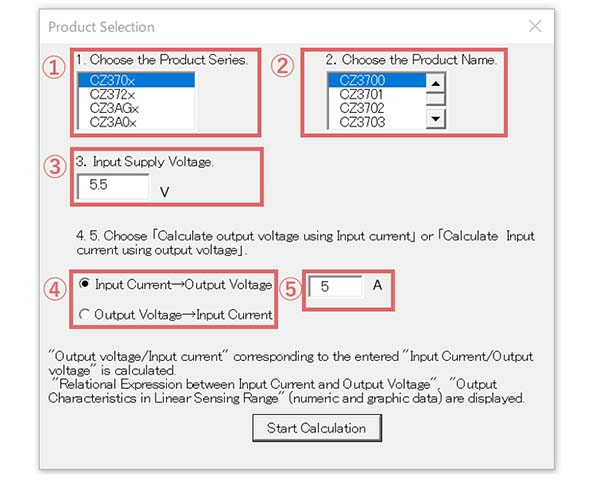Figure 1. Input form screen

1. Choose the product series.

2. Choose the brand of the product.

3. Input the Supply voltage.

* This option is available for ratiometric products only. If you select a non-ratiometric product, it will not be displayed because it does not depend on the supply voltage.

4. Choose one of the following options.

Input current → Output voltage / Output voltage → Input current

*If you select "Input current → Output voltage", you can check the output voltage value when a desired current value is input.

*If you select "Output voltage → Input current", you can check the input current value when a desired output voltage value is obtained.

5. Input current or output voltage

※Enter the "desired input current value" or a "desired output voltage value" that was selected in 4.

When you have completed the above input, click the Start Calculation button.

## Confirmation of simulation results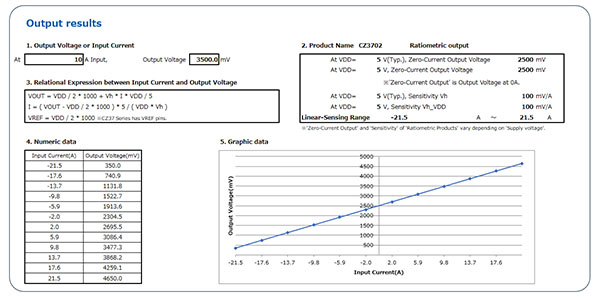Figure 2. Output Result Display Screen

When you click the Start Calculation button, the following results will be output.

・Output voltage or input current (calculated from the conditions that were selected and entered in 4 and 5 above)

・Product name

・Ratiometric / Non-ratiometric

・Zero current output voltage

・Sensitivity

・Linear Sensing Range

・Relational Expression between Input Current and Output Voltage

・Output Characteristics in Linear Sensing Range (numeric and graphic data)

This tool should be used on your PC.

## What is a ratiometric characteristic ?

There are two types of current sensor outputs: ratiometric and non-ratiometric. Please choose the one that best suits your situation.

### Ratiometric

Ratiometric : Zero current output voltage Vof and sensitivity Vh change in proportion to power supply voltage VDD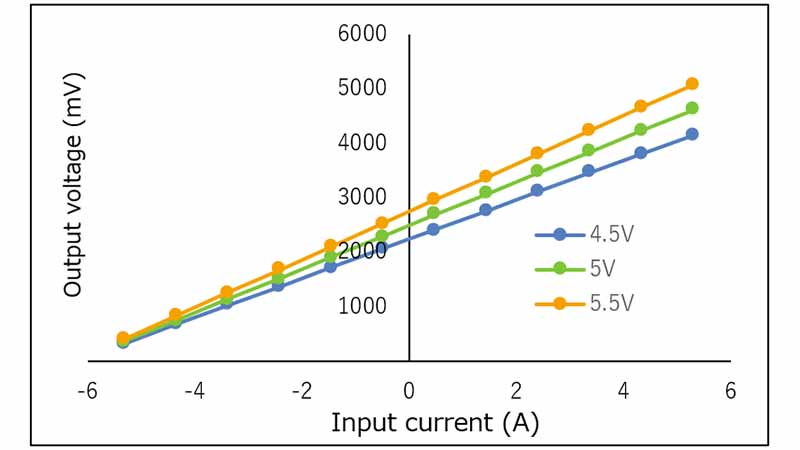Figure 3. Output of ratiometric product

Ratiometric products are suitable for the applications where power supplies for current sensors and measurement components such as ADCs can be standardized.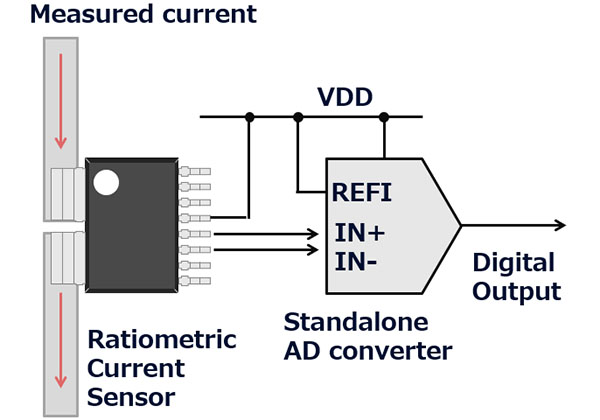Power supply swing can be offset by generating the ADC reference voltage VREFI from a common power supply.

Example)

The zero-current voltage (output voltage at 0A current) of the CZ375, CZ372, CZ370 series ratiometric product is 2.5V when the supply voltage is 5V.

Assume that 1LSB of ADC = VREFI / 1024. When the supply voltage is 5V and the current is 0A, the output is as follows: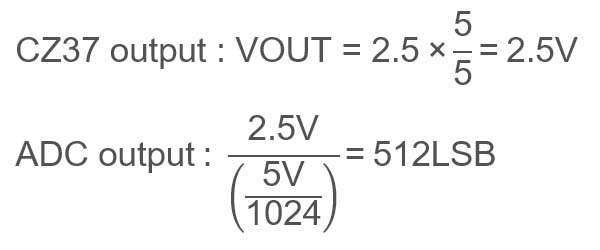When the supply voltage changes from 5V to 5.5V and the current is 0A, the output is as follows: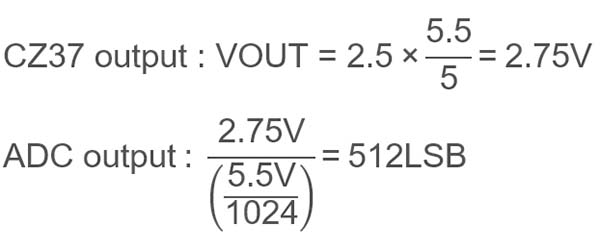As indicated above, by choosing a ratiometric product, the power supply dependency of the digital output can be eliminated.

Ratiometric products are suitable for current detection in areas that require accuracy, such as AC servos and the AC side of PV inverters.

### Non-ratiometric

Non-ratiometric : Output does not change even if power supply voltage VDD changes.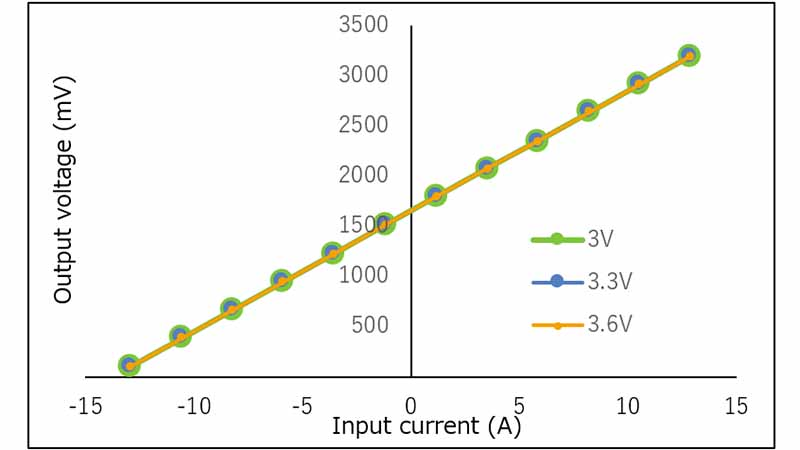Figure 4. Output for non-ratiometric products

Non-ratiometric products are suitable when the power supply for the current sensor and the measurement unit such as ADC cannot be standardized, such as when using an ADC with a built-in microcomputer.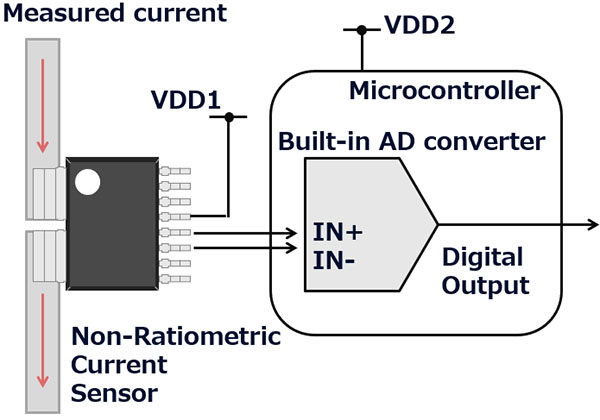This method achieves high accuracy by suppressing the supply voltage errors in both the current sensor and ADC.

AKM's current sensor suppresses the supply voltage error with the internal regulator. Its high PSRR makes it possible to reduce the power supply dependency even if the power supplies cannot be standardized, achieving high accuracy application.